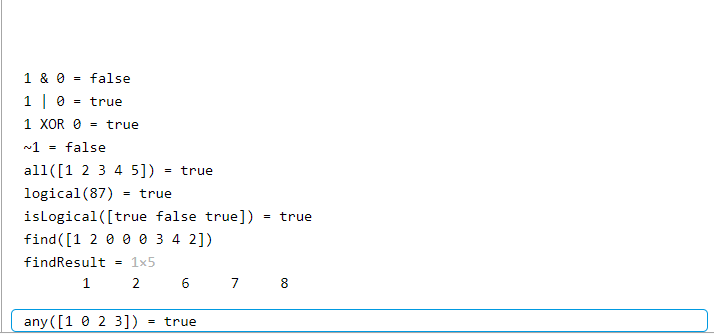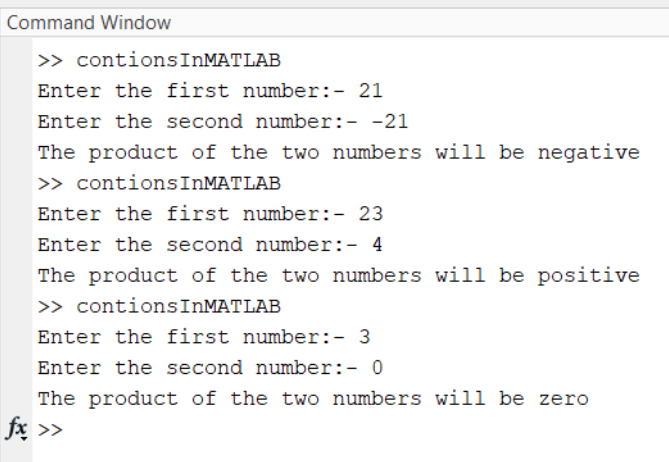Open in App
Not now

# How to Use Logical Operator Within If Statements in MATLAB?

• Last Updated : 30 Jun, 2022

Logical Operators are used to combining two or more conditions/constraints or to complement the evaluation of the original condition in consideration. The result of the operation of a logical operator is a boolean value either true or false. Like any other programming language, logical operators in MATLAB are beneficial, and in this article, we will demonstrate one of its uses.

## MATLAB logical operator and function:

• Element-wise: These operators function on corresponding factors of logical arrays. Element-wise logical operators function detail-by-detail on logical arrays. The symbols &, |, and ~ are the logical array operators AND, OR, and NOT.
• Short-circuit: These operators function on scalar, logical expressions. Short-circuit logical operators permit short-circuiting on logical operations. The symbols && and || are the logical short-circuit operators AND and OR.

But before we learn how to use logical operators with conditional statements, we should have a quick look at logical operators.

• Logical AND(&): True if both the operands are true.
• Logical OR( | ): True if either of the operands is true.
• Logical XOR(xor): The result of XOR is true if the two bits are different.
• Logical NOT( ~ ): True if the operand is false.

Now, here is a MATLAB example that illustrating logical operators with conditional statements.

Example 1:

## Matlab

 `% MATLAB code for Logicl Operators``% in use, 1 - True,  0 - False``% logical and operator``andResult = 1 & 0;` `% logical or operator``orResult = 1 | 0; ` `% logical xor operator``xorResult = xor(1,0);` `% logical not operator``notResult = ~1; ` `% logical all()``allResult = all([1 2 3 4 5]);` `% logical()``logicalResult = logical(76);` `% islogical()``isLogicalResult = islogical([true false true]);` `% logical find()``findResult = find([1 2 0 0 0 3 4 2]);` `% logical any()``anyResult = any([1 0 2 3]);` `disp(``"1 & 0 = "` `+ andResult);``disp(``"1 | 0 = "` `+ orResult);``disp(``"1 XOR 0 = "` `+ xorResult);``disp(``"~1 = "` `+ notResult);``disp(``"all([1 2 3 4 5]) = "` `+ allResult);``disp(``"logical(87) = "` `+ logicalResult);``disp(``"isLogical([true false true]) = "` `+ isLogicalResult);``disp(``"find([1 2 0 0 0 3 4 2])"``);``findResult``disp(``"any([1 0 2 3]) = "` `+ anyResult);`

Output:So now that we have a rudimentary understanding of the logical operator, we can use them in our conditional statements. Now we are going to use the logical operators in conditional statements.

Example 2:

## Matlab

 `% MATLAB script is used to determine``% the nature of the product (positive,``% negative or zero) of  the two``% numbers given by the user``num1 = input(``'Enter the first number:- '``);``num2 = input(``'Enter the second number:- '``);` `% here we are using && instead of & and ||``% instead of | because it makes the code``% execution faster as if the condition fails``% in the first operand the second operand is not tested``% If the both the numbers are greater that``% zero or both the numbers are less than zero``% their product will be positive``if``((num1 > 0 && num2 > 0) || (num1 < 0 && num2 < 0))``disp(``'The product of the two numbers will be positive'``);` `% If the numbers have opposite sign then``% their product will be negative``elseif``((num1 > 0 && num2 < 0) || (num1 < 0 && num2 > 0))``disp(``'The product of the two numbers will be negative'``);``else``disp(``'The product of the two numbers will be zero'``);``end`

Output:The above MATLAB script outputs the nature of the product of the two numbers given by the user and, while doing so, also illustrates how to use logical operators in conditional statements(like the If statement) So, logical operators are convenient when working with conditional statements.

## MATLAB functions for logical operations:

• all(): This determines if all array elements are nonzero or true. In this logical operation if all the elements of the array are non-zero then the output will be 1 (true) and If at least one of the elements is zero then the output will be 0 (false).
• logical(): This function converts the non-zero elements to 1 and the zero elements to 0.
• isLogical(): In this function If the input is logical i.e. true or false then the output will be 1, and if the input is anything apart from logical then the output will be 0.
• find(): Finds and returns the indices of non-zero elements in the array.
• any(): If any element in an array is non-zero the output is 1 if not then the output is 0.

My Personal Notes arrow_drop_up